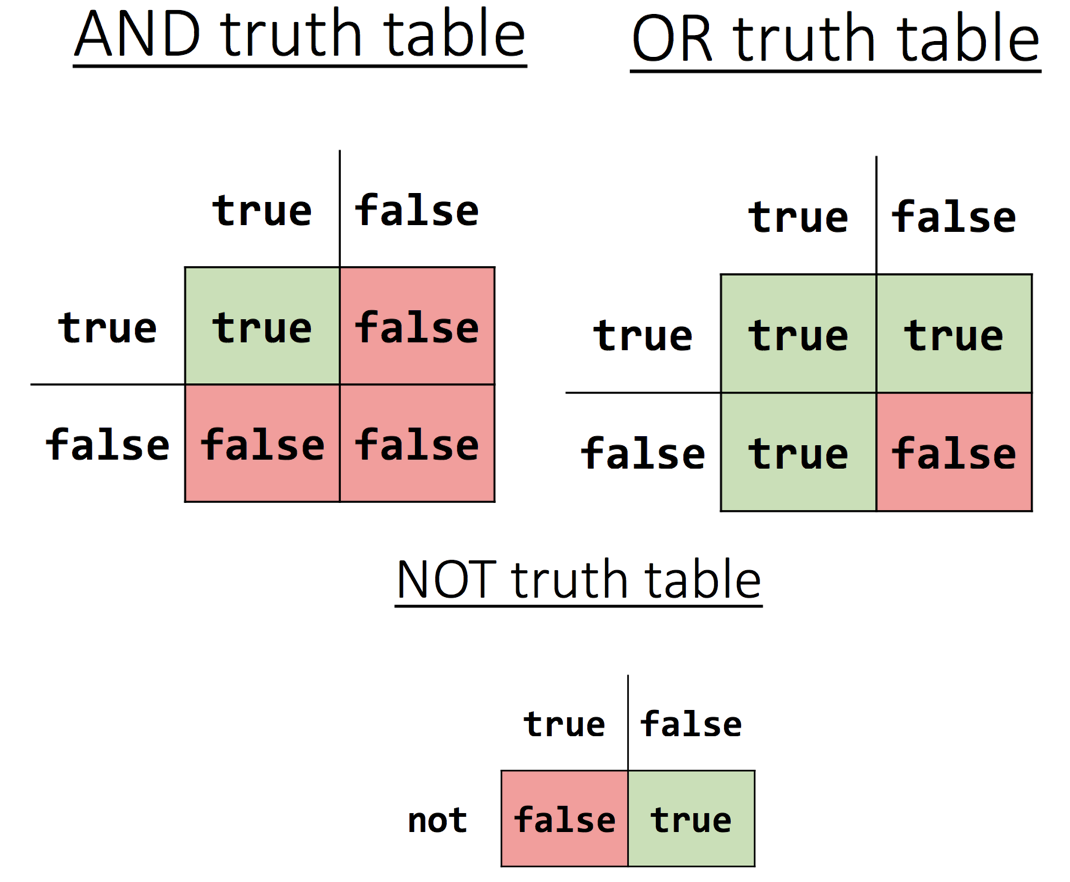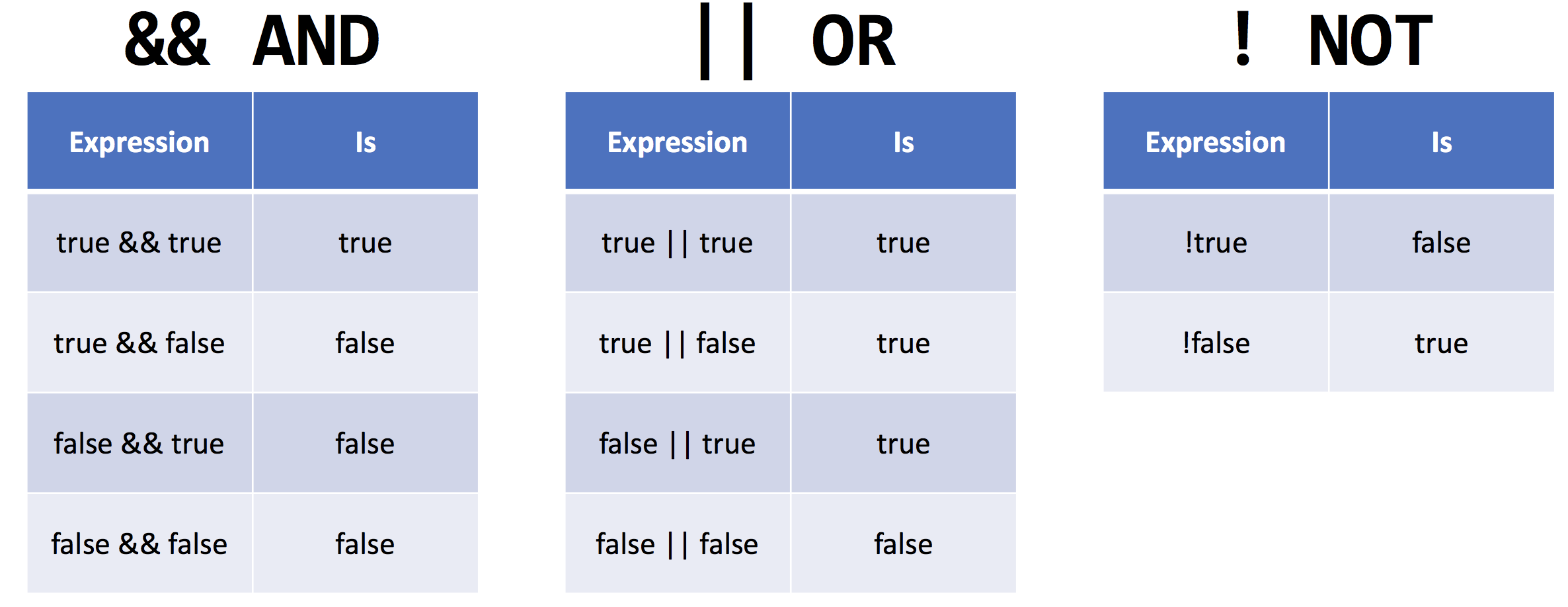#Comp 110 Expressions, Operators, and PEMDAS

## Expressions, Operators and PEMDAS

### Expressions

Expressions are combinations of variables, literals, operators, or functions that can be evaluated to produce a result. Expressions simplify to a single value.

For example:

```let z = y - x;
let torf = 2 > 5;
let six = 2 * 3;```

### Operators

Operators are special symbols to perform mathematical functions, comparisons, and assignments.

Most mathematical operators are exactly the same as they are in math! For example: +, -, / and * for addition, subtraction, division and multiplication.

However there are a few you may not be as familiar with. To raise a number to a power we use the double star (**).

`let x = 3**2; `

In the example above x would evaluate to 9.

Another less familiar operator is the modulus (%). The modulus divides one number by another and tells us the remainder.

`let x = 3%2;`

In the example above x would evaluate to 1, since two can go into three once, leaving a remainder of one.

For assigning variables, use the = operator, and the + for string concatenation.

```let firstName = "Creed";
let lastName = "Bratton";
let name = firstName + " " + lastName;```

In the above example we use the = operator to assign the variables firstName, lastName, and name values and use the + operator to concatenate firstName and lastName.

### PEMDAS

Feeling nostalgic for those 5th grade math classes? Well then Christmas has come early... PEMDAS is back!

IMPORTANT NOTE ABOUT COMP110: We assume that you have a solid foundation in algebraic problem solving! If you are concerned about your understanding of algebraic concepts, check out some Khan Academy tutorials or use the Message My Team function and reach out to your TAs about your concerns!

It works the same as when you learnt it all that time ago, but as a quick reminder:

P - Parentheses

E - Exponents

M - Multiplication

D - Division

S - Subtraction

So, for example,

4 + 5 * (18 / 3) - 4 could be broken down step by step:

parentheses (18 / 3) = 6,

followed by the multiplication, 5 * 6 = 30,

ending with addition and subtraction from left to right, 4 + 30 - 4 = 30.

### Boolean Expressions

Boolean Expressions

Booleans are either true or false, but we can also have expressions that are true or false. This means we can have whole expressions which are actually just booleans?

What is this sorcery?! Actually it's quite simple, and unfortunately involves no sorcery at all; they're called conditional operators. There are several operators that help compare data, and we'll discuss them below.

### Relational Operators

A lot of these symbols come to us from math and are pretty recognizable:

>

• This is the 'greater than' operator
• "Is the number on the left of the > larger than the one on the right?"
• If yes -> true
• If no -> false

<

• This is the 'less than' operator
• "Is the number on the left of the < smaller than the one on the right?"
• If yes -> true
• If no -> false

>=

• This is the 'is at least' operator, and looks like ≥ in maths
• "Is the number on the left of the  >= greater than or equal to the one on the right?"
• If yes -> true
• If no -> false

<=

• This is the 'is at most' operator, and looks like ≤ in maths
• "Is the number on the left of the <= less than or equal to the one on the right?
• If yes -> true
• If no -> false

Now that's all well and good, but let's look at them in some code!

``````let x = 31;
let y = 41;
let z = 41;

// below we'll test each of the operators and assign a boolean to the outcome

let booleanGreater = x > y;
print(booleanGreater); //prints true, because x > y in this case is 31 > 41, which is false, because 31 is less, not greater than 41

let booleanLess = x < y;
print(booleanLess); // prints true, because x < y in this case is 31 < 41, which is true. 31 is less than 41

let booleanAtLeast = y >= z
print(booleanAtLeast) // prints true, because even though y is not greater than z, it is equal to it, and therefore the expression will be true

let booleanAtMost = z <= x;
print(booleanAtMost); // prints false, because z is not less than or equal to x

``````

### Useful Operators for Loops

There are two special operators that are especially useful when writing loops. These are the ++ and -- operators.

The ++ operator is used to add 1 to a number variable, and the -- subtracts one from a number variable.

i++ is the same as writing i = i + 1

i-- is the same as writing i = i - 1

An example using a while loop is shown below

``````while(i < 10) {
// loop body
i++;
}``````

### Equality Operators

As it turns out, the = symbol does more fancy tricks than just assign variables! The following operators are used to check whether something is equal to something else:

===

• This is the equal to operator. It has THREE equals symbols in a row.
• It should only be used with simple data types (number, string, and boolean)
• eg
• 1066 === 1066 would be true
• 21 === 753 would be false

!==

• This is the not equal to operator. It uses the ! symbol to mean "NOT."
• It is the ! symbol followed by TWO equals symbols.
• eg
• 1066 !== 1066 would be false
•  21 !== 753 would be true

We'll see them in practice below

``````let x = 12;
let y = 13;

let b = x === y;
print(b); // prints false, because x (12) is not equal to y (13)

let d = x !== y;
print(d); // prints true, because x (12) is not equal to y (13)``````

### Logical Operators

Logical operators and negation are useful for creating compound logical statements.

• &&
• AND operator
• double ampersand
• Using the AND operator with boolean expressions results in true only if both expressions are true.
• ||
• OR operator
• double vertical bar
• Using the OR operator with boolean expressions results in true if either expression is true or if both expressions are true.
• !
• NOT operator
• This operator gives us the flipped meaning of a boolean - so if something normally evaluates to true, prefacing it with the ! operator will result in a false value, and if something is normally false, using the ! operator will result in true.

Here are some examples putting everything we've learned together!

``````let x: number = 7;
let y: number = 3;

let a: boolean = x > y;
print(a); //prints true
print(!a); //prints false - the inverse of a

let b: boolean = y >= x;
print(b); //prints false

let c: boolean = a && b;
print(c); //prints false - remember for && both a and b must return true

let d: boolean = a || b;
print(d); //prints true - for || only one must be true for the statement to return true``````

### Operation Assignment Operators

Modifying the value stored in a variable, then storing the modified version back in the variable is pretty common, so there are operators designed to make this easier for us!

There are operators for things like modifying numbers, whether by addition, multiplication, or any of the other arithmetic operations. There is also an operation assignment operator for concatenation! Here's a list:

• +=
• x += 110;
• same as: x = x + 110;
• -=
• subtraction assignment
• x -= 5;
• same as: x = x - 5;
• *=
• multiplication assignment
• x *= 6;
• same as: x = x * 6;
• /=
• division assignment
• x /= 4;
• same as: x = x / 4;
• %=
• modulo assignment
• x %= 2;
• same as: x = x % 2;
• **=
• exponentiation assignment
• x **= 3;
• same as: x = x ** 3;
• +=
• concatenation assignment
• x += "a";
• same as: x = x + "a";

Here's an example:

``````let num = 1;
let str = "comp";
num += 3; // num is now 4
num -= 2; // num is now 2
num *= 5; // num is now 10
num /= 2; // num is now 5
num %= 3; // num is now 2
num **= 3; // num is now 8
num += 102; // num is now 110
str += num; // str is now "comp110"``````

### Useful Tables